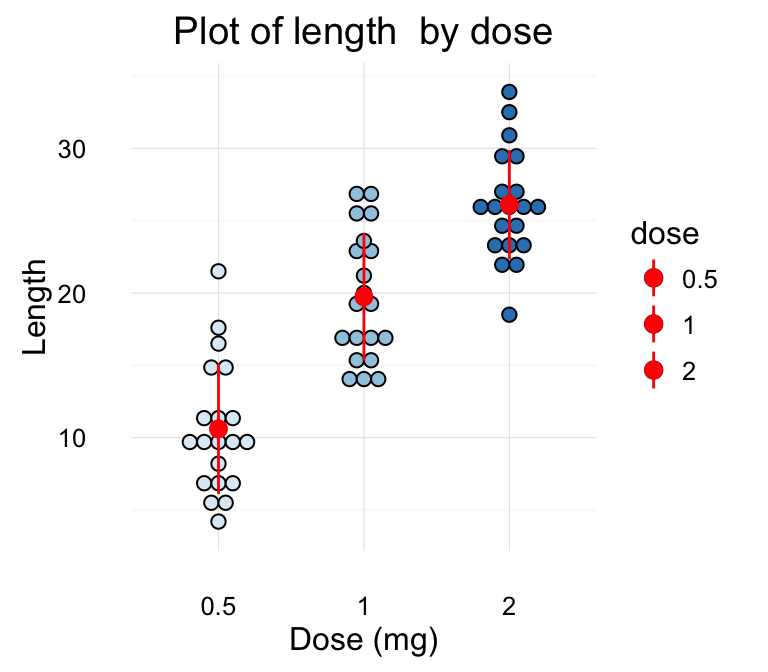# ggplot2 dot plot : Quick start guide - R software and data visualization

This R tutorial describes how to create a dot plot using R software and ggplot2 package.

The function geom_dotplot() is used.# Prepare the data

ToothGrowth data sets are used :

``````# Convert the variable dose from a numeric to a factor variable
ToothGrowth\$dose <- as.factor(ToothGrowth\$dose)
``````##    len supp dose
## 1  4.2   VC  0.5
## 2 11.5   VC  0.5
## 3  7.3   VC  0.5
## 4  5.8   VC  0.5
## 5  6.4   VC  0.5
## 6 10.0   VC  0.5``````

Make sure that the variable dose is converted as a factor variable using the above R script.

# Basic dot plots

``````library(ggplot2)
# Basic dot plot
p<-ggplot(ToothGrowth, aes(x=dose, y=len)) +
geom_dotplot(binaxis='y', stackdir='center')
p
# Change dotsize and stack ratio
ggplot(ToothGrowth, aes(x=dose, y=len)) +
geom_dotplot(binaxis='y', stackdir='center',
stackratio=1.5, dotsize=1.2)
# Rotate the dot plot
p + coord_flip()``````Choose which items to display :

``p + scale_x_discrete(limits=c("0.5", "2"))``# Add summary statistics on a dot plot

The function stat_summary() can be used to add mean/median points and more to a dot plot.

## Add mean and median points

``````# dot plot with mean points
p + stat_summary(fun.y=mean, geom="point", shape=18,
size=3, color="red")
# dot plot with median points
p + stat_summary(fun.y=median, geom="point", shape=18,
size=3, color="red")``````## Dot plot with box plot and violin plot

``````# Add basic box plot
ggplot(ToothGrowth, aes(x=dose, y=len)) +
geom_boxplot()+
geom_dotplot(binaxis='y', stackdir='center')
ggplot(ToothGrowth, aes(x=dose, y=len)) +
geom_boxplot(notch = TRUE)+
geom_dotplot(binaxis='y', stackdir='center')
ggplot(ToothGrowth, aes(x=dose, y=len)) +
geom_violin(trim = FALSE)+
geom_dotplot(binaxis='y', stackdir='center')``````Read more on box plot : ggplot2 box plot

Read more on violin plot : ggplot2 violin plot

## Add mean and standard deviation

The function mean_sdl is used. mean_sdl computes the mean plus or minus a constant times the standard deviation.

In the R code below, the constant is specified using the argument mult (mult = 1). By default mult = 2.

The mean +/- SD can be added as a crossbar or a pointrange :

``````p <- ggplot(ToothGrowth, aes(x=dose, y=len)) +
geom_dotplot(binaxis='y', stackdir='center')
p + stat_summary(fun.data="mean_sdl", fun.args = list(mult=1),
geom="crossbar", width=0.5)
p + stat_summary(fun.data=mean_sdl, fun.args = list(mult=1),
geom="pointrange", color="red")``````Note that, you can also define a custom function to produce summary statistics as follow.

``````# Function to produce summary statistics (mean and +/- sd)
data_summary <- function(x) {
m <- mean(x)
ymin <- m-sd(x)
ymax <- m+sd(x)
return(c(y=m,ymin=ymin,ymax=ymax))
}``````

Use a custom summary function :

``p + stat_summary(fun.data=data_summary, color="blue")``# Change dot plot colors by groups

In the R code below, the fill colors of the dot plot are automatically controlled by the levels of dose :

``````# Use single fill color
ggplot(ToothGrowth, aes(x=dose, y=len)) +
# Change dot plot colors by groups
p<-ggplot(ToothGrowth, aes(x=dose, y=len, fill=dose)) +
geom_dotplot(binaxis='y', stackdir='center')
p``````It is also possible to change manually dot plot colors using the functions :

• scale_fill_manual() : to use custom colors
• scale_fill_brewer() : to use color palettes from RColorBrewer package
• scale_fill_grey() : to use grey color palettes
``````# Use custom color palettes
p+scale_fill_manual(values=c("#999999", "#E69F00", "#56B4E9"))
# Use brewer color palettes
p+scale_fill_brewer(palette="Dark2")
# Use grey scale
p + scale_fill_grey() + theme_classic()``````Read more on ggplot2 colors here : ggplot2 colors

# Change the legend position

``````p + theme(legend.position="top")
p + theme(legend.position="bottom")
p + theme(legend.position="none") # Remove legend``````The allowed values for the arguments legend.position are : “left”,“top”, “right”, “bottom”.

Read more on ggplot legends : ggplot2 legend

# Change the order of items in the legend

The function scale_x_discrete can be used to change the order of items to “2”, “0.5”, “1” :

``p + scale_x_discrete(limits=c("2", "0.5", "1"))``# Dot plot with multiple groups

``````# Change dot plot colors by groups
ggplot(ToothGrowth, aes(x=dose, y=len, fill=supp)) +
geom_dotplot(binaxis='y', stackdir='center')
# Change the position : interval between dot plot of the same group
p<-ggplot(ToothGrowth, aes(x=dose, y=len, fill=supp)) +
geom_dotplot(binaxis='y', stackdir='center',
position=position_dodge(0.8))
p``````Change dot plot colors and add box plots :

``````# Change colors
p+scale_fill_manual(values=c("#999999", "#E69F00", "#56B4E9"))
ggplot(ToothGrowth, aes(x=dose, y=len, fill=supp)) +
geom_boxplot(fill="white")+
geom_dotplot(binaxis='y', stackdir='center')
# Change the position
ggplot(ToothGrowth, aes(x=dose, y=len, fill=supp)) +
geom_boxplot(position=position_dodge(0.8))+
geom_dotplot(binaxis='y', stackdir='center',
position=position_dodge(0.8))``````# Customized dot plots

``````# Basic dot plot
ggplot(ToothGrowth, aes(x=dose, y=len)) +
geom_boxplot()+
geom_dotplot(binaxis='y', stackdir='center')+
labs(title="Plot of length  by dose",x="Dose (mg)", y = "Length")+
theme_classic()
# Change color by groups
dp <-ggplot(ToothGrowth, aes(x=dose, y=len, fill=dose)) +
geom_dotplot(binaxis='y', stackdir='center')+
labs(title="Plot of length  by dose",x="Dose (mg)", y = "Length")
dp + theme_classic()``````Change fill colors manually :

``````# Continuous colors
dp + scale_fill_brewer(palette="Blues") + theme_classic()
# Discrete colors
dp + scale_fill_brewer(palette="Dark2") + theme_minimal()
dp + scale_fill_brewer(palette="RdBu") + theme_minimal()``````Read more on ggplot2 colors here : ggplot2 colors

# Infos

This analysis has been performed using R software (ver. 3.2.4) and ggplot2 (ver. 2.1.0)

<!-- END HTML -->